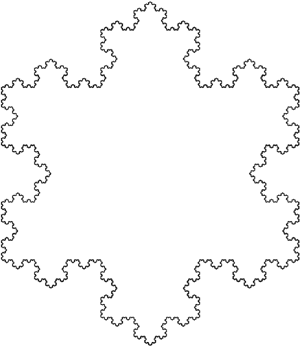A# Koch snowflakeThe Koch snowflake is one of the most symmetric and easy to understand fractals. It is named after the Swedish mathematician Helge von Koch (1870–1924), who first described it in 1906.

To make the snowflake, start with a straight line and split it into three equal parts. Replace the middle part with two lines, both of the same length as the first three, creating a equilateral triangle missing the bottom line. The shape now consists of four straight lines with the same length. For each of these lines, repeat the process above, then continue the transformation indefinitely. The Koch curve has infinite length because each iteration increases the length of a line segment one third, and the iterations go on forever. The same kind of process can be applied to a tetrahedron. Take a regular tetrahedron (all side lengths the same) and glue to each of its faces a smaller regular tetrahedron (Each smaller tetrahedron is scaled down by a factor of ½ from the larger one, and placed on each face in an inverted fashion, so that it divides the face into four equilateral triangles and covers the center one.) Then iterate this process. Intuition suggests that the end product might be a very strange-looking jagged object. But in fact, in the limit, as the number of iterations tends to infinity, the result is a perfect cube! The cube has side length t/√2, where t is the length of one of the edges of the regular tetrahedron you started with.

Variations on the flat Koch snowflake include the so-called exterior snowflake, the Koch antisnowflake, and the flowsnake curves.